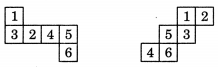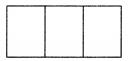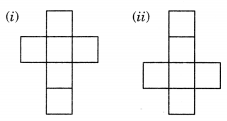# NCERT Solutions for Class 7 Maths Chapter 15 Visualising Solid Shapes

NCERT Solutions for Class 7 Maths Chapter 15 Visualising Solid Shapes

### NCERT Solutions for Class 7 Maths Chapter 15 Visualising Solid Shapes Ex 15.1

NCERT Solutions for Class 7 Maths Chapter 15 Visualising Solid Shapes Exercise 15.1

Ex 15.1 Class 7 Maths Question 1.
Identify the nets which can be used to make cubes (Cut out copies of the nets and try it):Solution:
Fig. (ii), (iii), (iv) and (vi) can be used to make cubes.

Ex 15.1 Class 7 Maths Question 2.
Dice are cubes with dots on each face. Opposite faces of a die always have a total of seven dots on them.
Here are two nets to make dice (cubes); the number inserted in each square indicate the number of dots in that box.Insert suitable number in the blanks, remembering that the number on the opposite faces should total to 7.
Solution:Ex 15.1 Class 7 Maths Question 3.Solution:
If one pair of opposite faces will have 1 and 4 on them, then their total does not come 7.
If we take 3 and 6 on the opposite faces of the die, then the total does not come 7.
Hence, this cannot be for a die.

Ex 15.1 Class 7 Maths Question 4.
Here is an incomplete net for making a cube. Complete it in atleast two different ways. Remember that a cube has six faces. How many are there in the net here? (Give two separate diagrams. If you like, you may use a squared sheet for easy manipulation).Solution:
There are three faces in the given net.Ex 15.1 Class 7 Maths Question 5.
Match the nets with appropriate solids:Solution:
(a) (ii)
(b) (iii)
(c) (iv)
(d) (i)## SabDekho

The Complete Educational Website